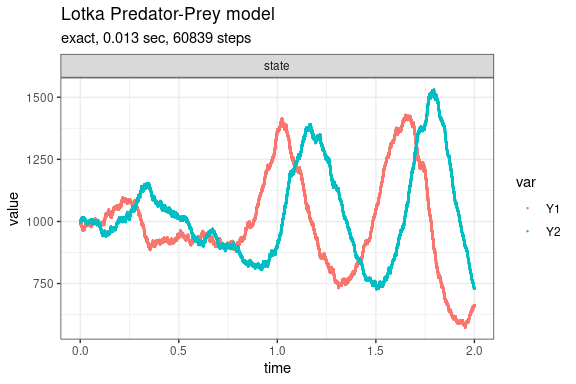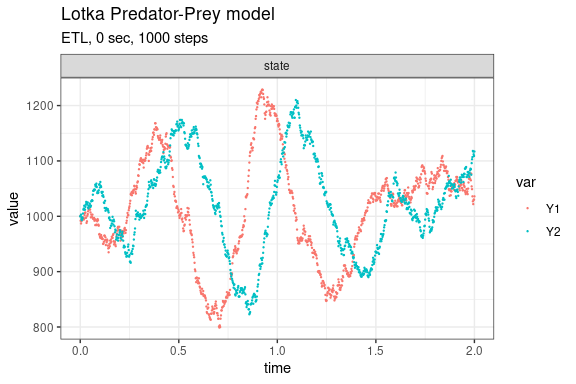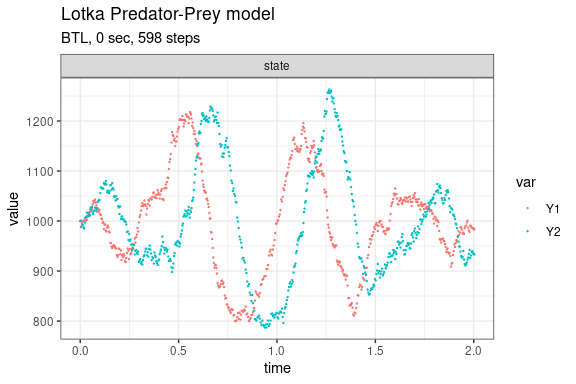# Lotka Predator-Prey model (Gillespie, 1977; Kot, 2001)

This version of the Lotka predator-prey model is given by

dY1/dt = c1*Y1 - c2*Y1*Y2
dY2/dt = c2*Y1*Y2 - c3*Y2

consisting of the three reaction channels,

      Y1 --c1--> Y1 + Y1
Y1 + Y2 --c2--> Y2 + Y2
Y1 --c3--> 0

Define parameters

library(GillespieSSA2)
sim_name <- "Lotka Predator-Prey model"
params <- c(c1 = 10, c2 = .01, c3 = 10)
final_time <- 2
initial_state <- c(Y1 = 1000, Y2 = 1000)

Define reactions

reactions <- list(
reaction("c1 * Y1", c(Y1 = +1)),
reaction("c2 * Y1 * Y2", c(Y1 = -1, Y2 = +1)),
reaction("c3 * Y2", c(Y2 = -1))
)

Run simulations with the Exact method

set.seed(1)
out <- ssa(
initial_state = initial_state,
reactions = reactions,
params = params,
final_time = final_time,
method = ssa_exact(),
sim_name = sim_name
)
autoplot.ssa(out)Run simulations with the Explict tau-leap method

set.seed(1)
out <- ssa(
initial_state = initial_state,
reactions = reactions,
params = params,
final_time = final_time,
method = ssa_etl(tau = .002),
sim_name = sim_name
)
autoplot.ssa(out)Run simulations with the Binomial tau-leap method

set.seed(1)
out <- ssa(
initial_state = initial_state,
reactions = reactions,
params = params,
final_time = final_time,
method = ssa_btl(mean_firings = 100),
sim_name = sim_name
)
autoplot.ssa(out)